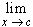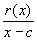#Interactive Real Analysis

Next | Previous | Glossary | Map

## 6.5. Differentiable Functions

### Theorem 6.5.3: Derivative as Linear Approximation

Let f be a function defined on (a, b) and c any number in (a, b). Then f is differentiable at c if and only if there exists a constant M such that
f(x) = f(c) + M ( x - c ) + r(x)
where the remainder function r(x) satisfies the condition= 0

### Proof:

First, suppose f is differentiable at x = c. Let the constant M = f'(c) and set

• r(x) = f(x) - f(c) - f'(c) ( x - c )

We have to check the limit of the quotient

•=- f'(c)

Since f is differentiable, the limit of this expression is zero as x approaches c, as required.

Second, suppose that f(x) = f(c) + M ( x - c ) + r(x) for some constant M and= 0 Then

•- M =The limit on the right as x approaches c is zero by assumption. Hence, the limit on the left must also be zero, and we recognize the constant M as the derivative of the function f'(c).Next | Previous | Glossary | Map